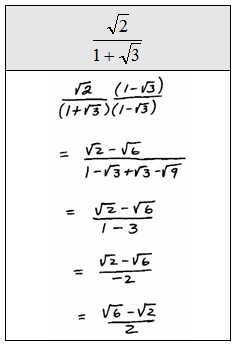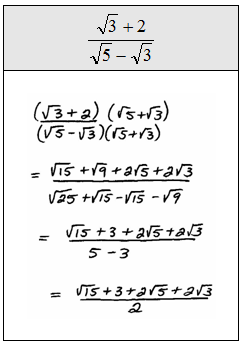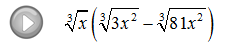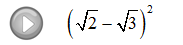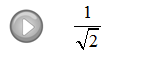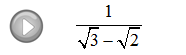## Pages

### Multiplying and Dividing Radical Expressions

As long as the indices are the same, we can multiply the radicands together using the following property.Since multiplication is commutative, you can multiply the coefficients and the radicands together and then simplify.

Multiply.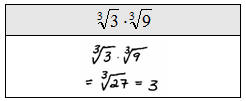Take care to be sure that the indices are the same before multiplying.  We will assume that all variables are positive.

Simplify.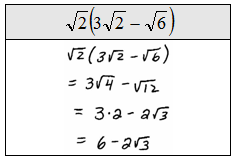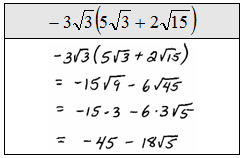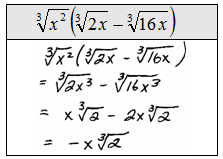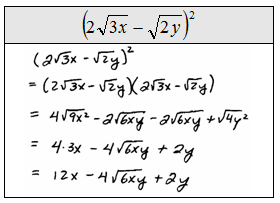Divide radicals using the following property.Divide. (Assume all variables are positive.)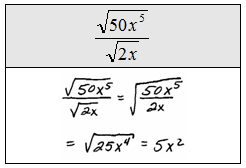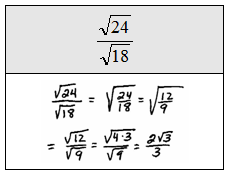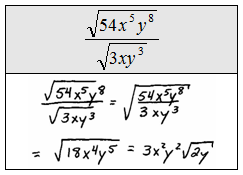Rationalizing the Denominator
A simplified radical expression cannot have a radical in the denominator.  When the denominator has a radical in it, we must multiply the entire expression by some form of 1 to eliminate it.  The basic steps follow.

Rationalize the denominator: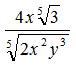Multiply numerator and denominator by the 5th root of of factors that will result in 5th powers of each factor in the radicand of the denominator.Notice that all the factors in the radicand of the denominator have powers that match the index.  This was the desired result, now simplify.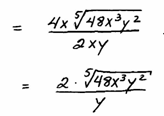Rationalize the denominator.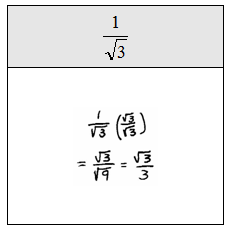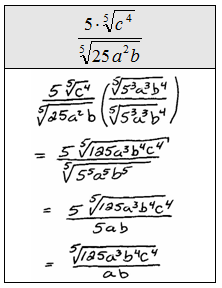This technique does not work when dividing by a binomial containing a radical.  A new technique is introduced to deal with this situation.

Rationalize the denominator:Multiply numerator and denominator by the conjugate of the denominator.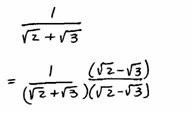And then simplify. The goal is to eliminate all radicals from the denominator.Rationalize the denominator.